## Chemistry Paper 1 Questions and Answers - Sukellemo Joint Mock 2020/2021

SUKELLEMO JOINT MOCK
Kenya Certificate of Secondary Education
CHEMISTRY PAPER 1
(THEORY)

2 hours

Instructions to Candidates

• Answer all the questions in the spaces provided.
• All working must be clearly shown.
• Non-programmable silent electronic calculators and KNEC mathematical tables may be used.
1. Explain how a burette is suited to its function of accurate measurement of volume of liquids(1 mark)
2. A mixture of two liquids A and B with densities of 2.34g/cm3 and 1.30g/cm3 respectively is to be separated .
1. Describe how to efficiently obtain liquid B from the mixture . (2 marks
2. Name the type of mixture consisting of liquids A and B (1 mark)
3.  Nitrogen (I)oxide gas can be prepared in the laboratory using a mixture of ammonium chloride solution and sodium nitrate solution.
1. The reaction occurs in two steps. State the two steps in the correct order. (2 marks)
2. State one use of nitrogen (I) oxide (1 mark)
4.
1. Draw structural formulae of two positional isomers with molecular formula C4H8.
2. Study the equation below and answer the questions that follow.
C4H8 + Cl2 → C4H8Cl2
1. State the condition under which this reaction occurs. (1 mark)
2. Give the general name of this type of reaction. (1 mark)
5. The diagram below shows the energy changes that occur when sodium chloride dissolves in water. Study it and answer the questions that follow.1. What do ∆H1, ∆H2 and ∆H3 represent? (1½ mark)
∆H1
∆H2
∆H3
2. Given that the lattice energy of NaCl (s) is -776 kJmol-1 and hydration energies of Na+(g) and Cl-(g) are -407 kJmol-1 and -364 kJmol-1 respectively. Calculate the heat of solution (∆Hsol) of 1 mole NaCl(s). (1½ mark)
6. The standard electrode potentials of a metal H and Iron are given below.
Fe2+(aq) + 2e →Fe(s) -0.44V
H2+(aq) + 2e →H(s) -0.91V
Is the reaction below possible ? Show your working (2 marks)
Fe(s) + H2+(aq) → H(s) + Fe2+(aq)
7.
1. Why is the percentage of carbon (IV) oxide in the atmosphere fairly constant? (1 mark)
2. Calculate the volume of carbon(IV)oxide in 9,000 m3 of air contained in a room. (2 marks)
8. State two applications of using impurities to lower the melting point of substances. (2 marks)
9.
1. Determine the percentage of nitrogen in ammonium nitrate fertilizer (N=14.0, H=1.0,0=16.0 ) (2 marks)
2. Give one negative effect of continuous use of nitrogenous fertilizers (1 mark)
10. Study the table below and use it to answer the questions that follow. (The letters do not represent the actual symbols of the elements).
 Element S P Q R T AtomicNumber 5 20 3 18 5 Atomic mass 10 40 7 40 11

1. Select two letters that represent a pair of isotopes ? (1 mark)
2. Give the number of neutrons in an atom of element T . (1 mark)
11. Dry ammonia gas was passed over heated copper(II) oxide.
1. Write an equation for the reaction. (1 mark)
2. Give the property of ammonia gas illustrated in the reaction above (1 mark
3. Write the formula of a gas that can be used in place of ammonia gas in the reaction above
12. Study the equation below and answer the questions that follow:1. What type of reaction is this? (1 mark)
2. What class of organic compounds does X belong? (1 mark)
3. Explain the role of sodium chloride in the manufacture of soap. (1 mark)
13. The set up below was used to prepare dry hydrogen gas. Study it and answer the questions that follow.1. Identify one mistake in the set-up above. (1 mark)
2. What is the role of Liquid Z? (1 mark)
3. Using an equation give one chemical property of hydrogen gas (1 mark)
14. Study the following equilibrium equation.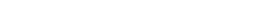1. Suggest two ways of increasing the yield of A2B. (1 mark)
2. Draw the energy level diagram for the backward reaction. (2 marks)
15. A solution contains 5.3g of X2CO3 in 500 cm3 of solution. 25.0 cm3 of this solution required 20.0 cm3 of 0.25M hydrochloric acid for complete neutralization.
The equation for the reaction is;
X2CO3 (aq) + 2HCl (aq) → 2XCl (aq) + H2O (l) + CO2 (g)
1. Calculate the concentration of X2CO3 solution in grams/dm3. (1 mark)
2. Find the molarity of X2CO3 solution. (1 mark)
3. Find the relative formula mass of X2CO3 hence calculate the relative atomic mass of X.
(C = 12, O = 16) (2 marks)
16.
1. State Graham’s law of diffusion.
2. 100cm3 of Carbon (IV) Oxide diffuses through a porous plate in 30 seconds. Calculate the time taken by 75cm3 of Nitrogen (IV) Oxide to diffuse through the same plate under similar conditions. (C = 12, 0 = 16, N = 14) (2 marks)
17. A student fetched water from a river in a limestone area. He used it for washing and realized that it did not lather easily. On boiling,the water lathered easily .When he added washing soda,it again lathered easily
1. Name two compounds that are most likely to be present in the water (1 mark)
2. Given that the structure of soap is C17H35COONa.
Explain by means of ionic equation how the above compounds prevent lathering. (1 mark)
18. A student burnt magnesium ribbon in a gas jar full of sulphur (IV) oxide gas.
1. State two observations made in the gas jar. (2 mark
2. Write an equation for the reaction that took place. (1 mark)
19. A radioactive isotope M decays by emitting two alpha and a beta particle to form 206 Y.
1. What was the atomic number of M (1 mark)
2. After 224 days 1/16 of mass of M remained. Determine the half-life of M. (2 marks)
3. State one use of radioactivity in agriculture. (1 mark)
20.
1. With the aid of a well labeled diagram, show that the almost colourless region of a non luminous flame consist of unburnt gases (1½ marks)
2. Highlight the steps followed when lighting a Bunsen burner. (1½ marks)
21. The diagram below shows an experiment involving chlorine water.1. Chlorine water is a mixture of two acids. Explain using a chemical equation. (1 mark)
2. State and explain the observation that was made after 24 hours. (2 marks)
3. Write an equation for the reaction that took place in (b) above. (1 mark)
22. Study the flow chart below and use it to answer the questions that follow.1. Identify substances L, P, Q and S. (2 marks)
L
P
Q
S
2. Write an ionic equation for the reaction between T and silver nitrate solution. (1 mark)
23.
1. Define solubility. (1mark
2. In an experiment to determine the solubility of solid W in water at 400c, the following results were obtained.
• Mass of empty evaporating dish = 36.2g
• Mass of evaporating dish + saturated solution = 52.4g.
• Mass of evaporating dish + dry solid W = 40.4g
Use this data to calculate the solubility of W at 400C . (2 marks)
24. In terms of structure and bonding, explain why propane (RMM=44 ) is a gas at room temperature while ethanol (RMM=46) is a liquid at room temperature (2 marks)
25. Explain why hard water flowing in lead pipes may be safer for drinking than soft water flowing in the same pipes. (2 marks)
26. Air was passed through reagents as shown below.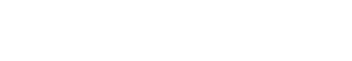1. State and explain the observations made when air is passed over hot copper. (2 marks)
2. Write the equation for the reaction taking place in chamber B (1 mark)
27. When sugar crystals were reacted with concentrated sulphuric (VI) acid, a black solid A was formed which when dried, burnt in excess air to form a colourless gas B only while when concentrated sulphuric (VI) acid is reacted with liquid C at a temperature of 170oC, a colourless gas D is formed which turns yellow bromine water to colourless and also turns colour of substance E from purple to colourless.
1. Identify substances : (2 marks)
Gas B
Liquid C
Gas D
2. Which property of concentrated sulphuric (VI) acid is being demonstrated by formation of the black solid (1 mark)
28. Sodium is extracted from its ore by the electrolysis process.
1. Name the chief ore from which sodium is extracted from. (1 mark)
2. An ore is suspected to contain mainly sodium. Describe a method that can be used to confirm the presence of sodium in the ore. (2 marks)

MARKING SCHEME

1. Explain how a burette is suited to its function of accurate measurement of volume of liquids
• It is narrow for small meniscus / It is long for smaller calibrations
2. A mixture of two liquids A and B with densities of 2.34g/cm3 and 1.30g/cm3 respectively is to be separated .
1. Describe how to efficiently obtain liquid B from the mixture . (2 marks)
• Place the mixture in a separating funnel ;allow it to settle.Open the tap and carefully let out the lower liquid A into a container . Discard the interphase .Liquid B remains in the separating funnel
2. Name the type of mixture consisting of liquids A and B (1 mark)
• Immiscible liquids
3. Nitrogen (I)oxide gas can be prepared in the laboratory using a mixture of ammonium chloride solution and sodium nitrate solution.
1. The reaction occurs in two steps. State the two steps in the correct order. (2 marks)
• Ammonium chloride and sodium nitrate solutions react to form ammonium nitrate and sodium chloride
• Ammonium nitrate solution undergoes thermal decomposition to for nitrogen (I) oxide and water
2. State one use of nitrogen (I) oxide (1 mark)
• Anaesthetic for dental surgery
• Produce flames for analytical work
• An oxidizer in racing car engines
4.
1. Draw structural formulae of two positional isomers with molecular formula C4H8.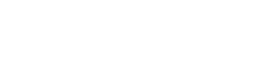2. Study the equation below and answer the questions that follow.
C4H8 + Cl2 → C4H8Cl2
1. State the condition under which this reaction occurs. (1 mark)
• Room temperature
2. Give the general name of this type of reaction. (1 mark)
5. The diagram below shows the energy changes that occur when sodium chloride dissolves in water. Study it and answer the questions that follow.1. What do ∆H1, ∆H2 and ∆H3 represent? (1½ mark)
∆H1 : Lattice energy
∆H2 : Hydration energy
∆H3 :Heat of solution
2. Given that the lattice energy of NaCl (s) is -776 kJmol-1 and hydration energies of Na+(g) and Cl-(g) are -407 kJmol-1 and -364 kJmol-1 respectively. Calculate the heat of solution (∆Hsol) of 1 mole NaCl (s). (1½ mark)
• (∆Hsol) = ∆H1 + ∆H2
= 776 + (-407-364) = + 5 kJmol-1
6. The standard electrode potentials of a metal H and Iron are given below.
Fe2+(aq) + 2e  → Fe(s) -0.44V
H2+(aq) + 2e  → H(s) -0.91V
Is the reaction below possible ? Show your working (2 marks)
Fe(s) + H2+(aq) → H(s) + Fe2+(aq)
• Emf = -0.91-( -0.44)= -0.47V
The reaction is not possible  since overall emf is negative
7.
1. Why is the percentage of carbon (IV) oxide in the atmosphere fairly constant? (1 mark)
• There is a balance between the processes that produce carbon (IV) oxide and processes that absorb it
2. Calculate the volume of carbon(IV)oxide in 9,000 m3 of air contained in a room. (2 marks)
• 9,000 x 0.03/100
= 2.7 m3
8. State two applications of using impurities to lower the melting point of substances. (2 marks)
• Extraction of metals from their molten compounds/ores
• Clearing of ice from roads in temperate countries
9.
1. Determine the percentage of nitrogen in ammonium nitrate fertilizer (N=14.0, H=1.0,0=16.0 ) (2 marks)
• RMM of NH4NO3 = 80
% N = 100 X 28/ 80 (1 mark) = 35 %
2. Give one negative effect of continuous use of nitrogenous fertilizers (1 mark)
• Causes eutrophication/rapid growth of water plants
• Make soil acidic
10. Study the table below and use it to answer the questions that follow. (The letters do not represent the actual symbols of the elements).
 Element S P Q R T AtomicNumber 5 20 3 18 5 Atomic mass 10 40 7 40 11

1. Select two letters that represent a pair of isotopes ? (1 mark)
• S and T  Mark as a pair
2. Give the number of neutrons in an atom of element T . (1 mark)
• 11- 5 = 6
11. Dry ammonia gas was passed over heated copper(II) oxide.
1. Write an equation for the reaction. (1 mark)
• 3 CuO (s) + 2 NH3 (g) → 3 Cu (s) + N2 (g) + 3 H2O (l)
2. Give the property of ammonia gas illustrated in the reaction above (1 mark
• Reducing property
3. Write the formula of a gas that can be used in place of ammonia gas in the reaction above
• CO / H2
12. Study the equation below and answer the questions that follow:1. What type of reaction is this? (1 mark)
• Hydrolysis
2. What class of organic compounds does X belong? (1 mark)
• Esters
3. Explain the role of sodium chloride in the manufacture of soap. (1 mark)
• Precipitates /hardens soap by lowering its solubility glycerol
13. The set up below was used to prepare dry hydrogen gas. Study it and answer the questions that follow.1. Identify one mistake in the set-up above. (1 mark)
• The method of gas collection
2. What is the role of Liquid Z? (1 mark)
• Absorbs moisture/water from hydrogen gas
3. Using an equation give one chemical property of hydrogen gas (1 mark)
• Burns in oxygen to form water
• 2H2 (g) + O2 (g)  → 2 H2O
• Removes oxygen from metals lower in the reactivity series
• H2 (g) + CuO(s) → Cu (s) + H2O (l)
14. Study the following equilibrium equation.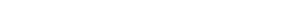1. Suggest two ways of increasing the yield of A2B. (1 mark)
• Lowering temperature
• Increasing pressure
2. Draw the energy level diagram for the backward reaction. (2 marks)15. A solution contains 5.3g of X2CO3 in 500 cm3 of solution. 25.0 cm3 of this solution required 20.0 cm3 of 0.25M hydrochloric acid for complete neutralization.
The equation for the reaction is;
• X2CO3 (aq) + 2HCl (aq)  → 2XCl (aq) + H2O (l) + CO2 (g)
1. Calculate the concentration of X2CO3 solution in grams/dm3. (1 mark)
• 5.3 g →500 cm3
?       →1000 cm3
? = 5.3 x1000 =  10.6g/ dm3
500
2. Find the molarity of X2CO3 solution. (1 mark)
• Moles of HCl = 20 x 0.25 =0.005
1000
• Moles of X2CO3 = 0.05 =0.0025
2
• Molarity of X2CO3= 0.0025 x 1000 = 0.1 M
25
3. Find the relative formula mass of X2CO3 hence calculate the relative atomic mass of X.(C = 12, O = 16) (2 marks)
• Formula mass = 10.6 = 106
0.1
• 2X +12+48 =106
• X=23 (1 mk)
16.
1. State Graham’s law of diffusion. (1 mark)
• At constant temperature and pressure, the rate of diffusion of a gas is inversely proportional to the square root of its density / molecular mass
2. 100cm3 of Carbon (IV) Oxide diffuses through a porous plate in 30 seconds. Calculate the time taken by 75cm3 of Nitrogen (IV) Oxide to diffuse through the same plate under similar conditions. (C = 12, 0 = 16, N = 14) (2 marks)
• 75cm3 of CO2 takes = (75 x 30)/100 second = 22.5 s
• Rmm of CO2 = 12 + 2 x 16 = 44
• Rmm of NO2 = 14 + 2 x 16 = 46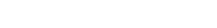•  = 23.006s
17. A student fetched water from a river in a limestone area. He used it for washing and realized that it did not lather easily. On boiling,the water lathered easily .When he added washing soda,it again lathered easily
1. Name two compounds that are most likely to be present in the water (1 mark)
• Calcium hydrogen carbonate
• Magnesium hydrogen carbonate
2. Given that the structure of soap is C17H35COONa.
Explain by means of ionic equation how the above compounds prevent lathering. (1 mark)
• C17H35COO- (aq ) + Ca2+(aq) → ( C17H35COO)2Ca (s)
18. A student burnt magnesium ribbon in a gas jar full of sulphur (IV) oxide gas.
1. State two observations made in the gas jar. (2 mark)
• A yellow powder of sulphur was deposited
• White solid of magnesium oxide is formed
2. Write an equation for the reaction that took place. (1 mark)
• 2Mg(s) + SO2(g) 2MgO(s) + S (s)
19. A radioactive isotope M decays by emitting two alpha and a beta particle to form 206 83Y.
1. What was the atomic number of M (1 mark)
• Atomic number = 83 + 4 -1 = 86
2. After 224 days 1/16 of mass of M remained. Determine the half-life of M. (2 marks)

•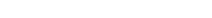There are 4 t½

4 t½ = 224
t½ = 56 days
3. State one use of radioactivity in agriculture. (1 mark)
• Monitoring uptake of nutrients such as phosphates by plants
1. With the aid of a well labeled diagram, show that the almost colourless region of a non luminous flame consist of unburnt gases (1½ marks)2. Highlight the steps followed when lighting a Bunsen burner. (1½ marks)
• Close the air-hole
• Strike a match and place it on top of the chimney
• Open the air-hole
20. The diagram below shows an experiment involving chlorine water.1. Chlorine water is a mixture of two acids. Explain using a chemical equation. (1 mark)
• Cl2 (g) + H2O (l) → HCl (aq) + HClO (aq)
2. State and explain the observation that was made after 24 hours. (2 marks)
• Yellow chlorine water turns colourless / Bubbles of a colourless gas  Chloric(I) acid decomposes in sunlight to form hydrochloric acid and oxygen gas
3. Write an equation for the reaction that took place in (b) above. (1 mark)
(sunlight)
• 2 HOCl (aq)    →   2 HCl (aq) + O2 (g)
21. Study the flow chart below and use it to answer the questions that follow.1. Identify substances L, P, Q and S. (2 marks)
• L - Calcium hydrogen carbonate / (CaHCO3)2
• P - Silver chloride / AgCl
• Q - Calcium carbonate / CaCO3
• S - Carbon (IV) oxide
2. Write an ionic equation for the reaction between T and silver nitrate solution. (1 mark)
• Ag+ (aq) + Cl- (aq) → AgCl (s)
22.
1. Define solubility. (1mark)
• The maximum mass of a solute that can dissolve in 100g of water at a given temperature
2. In an experiment to determine the solubility of solid W in water at 400c, the following results were obtained.
• Mass of empty evaporating dish = 36.2g
• Mass of evaporating dish + saturated solution = 52.4g.
• Mass of evaporating dish + dry solid W = 40.4g

Use this data to calculate the solubility of W at 400C .
• Mass of saturated solution = 52.4 - 36.2 = 16.2 g
• Mass of solid W = 40.4 – 36.2 = 4.2g
• Mass of water = 16.2 – 4.2 = 12g
• Solubility of solid W at 400c = 4.2 x 100 =  35g/100g of water
12
23. In terms of structure and bonding, explain why propane (RMM=44 ) is a gas at room temperature while ethanol (RMM=46) is a liquid at room temperature (2 marks)
• The molecules of propane are held together by weak Van der waals forces ;  whereas those of ethanol are held together by stronger hydrogen bonds
24. Explain why hard water flowing in lead pipes may be safer for drinking than soft water flowing in the same pipes. (2 marks)
• Formation of insoluble calcium carbonate/magnesium carbonate in hard water pipes prevents the lead pipes from dissolving into the water to cause lead poisoining
25. Air was passed through reagents as shown below.1. State and explain the observations made when air is passed over hot copper. (2 marks)
• The brown copper metal turns to black copper (II) oxide .  Copper is oxidized by oxygen to form copper (II)oxide
2. Write the equation for the reaction taking place in chamber B (1 mark)
• 3Mg (s) + N2(g) → Mg3N2 (s)
26. When sugar crystals were reacted with concentrated sulphuric (VI) acid, a black solid A was formed which when dried, burnt in excess air to form a colourless gas B only while when concentrated sulphuric (VI) acid is reacted with liquid C at a temperature of 170oC, a colourless gas D is formed which turns yellow bromine water to colourless and also turns colour of substance E from purple to colourless.
1. Identify substances : (2 marks)
• Gas B : Carbon (IV) oxide
• Liquid C : Ethanol
• Gas D : Ethene
• Substance E : Acidified potassium manganate (VII)
2. Which property of concentrated sulphuric (VI) acid is being demonstrated by formation of the black solid (1 mark)
• Dehydrating agent
27. Sodium is extracted from its ore by the electrolysis process.
1. Name the chief ore from which sodium is extracted from. (1 mark
• Rock salt
2. An ore is suspected to contain mainly sodium. Describe a method that can be used to confirm the presence of sodium in the ore. (2 marks)
• Crush the ore,add water and stir.  Filter and dip a glass rod in the filtrate.Remove the glass rod and heat in a non-luminous flame .Yellow flame confirms presence of sodium.

• ✔ To read offline at any time.
• ✔ To Print at your convenience
• ✔ Share Easily with Friends / Students